Related Tags

machine learning
community creator
data science
linear regression

# A deep dive into linear regression (3-way implementation)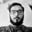Aman Anand

Linear Regression is the genesis of Machine Learning for many beginners. People start learning ML from Linear Regression and then go on to make awesome projects. If someone claims to be ignorant of Machine Learning’s awesomeness, they are surely living under a rock.

Let’s start with the basic concept of Machine Learning and take a tour through the world of statistics and Machine learning. Linear Regression basically means fitting a line for a set of points that represent the features.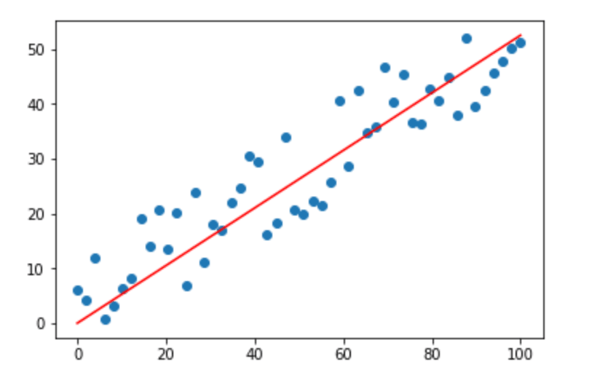Linear Regression is not only important for ML, it’s also important for Statistics. The method of Least square estimation is used in statistics to approximate the solution of linear regression by minimizing the least square distance of the points from the regression line.

The hypothesis function represents the equation of the line to be fitted. Here theta-0 and theta-1 represent the parameters of the regression line. In the line equation (y = mx + c), m is a slope and c is the y-intercept of the line. In the given equation, theta-0 is the y-intercept and theta-1 is the slope of the regression line.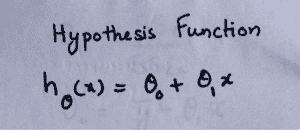Note: Here we are dealing with a single independent variable (x).

The cost function is the function we have to minimize to get the appropriate and optimum line. Here, the difference between h-theta and y is known as error. We take the mean of squared error as the cost function.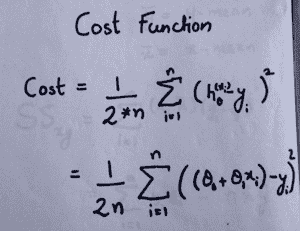The equations to calculate the value of theta-0 and theta-1 are given below. We calculate the values using these equations; this method is known as the Least Square estimation method.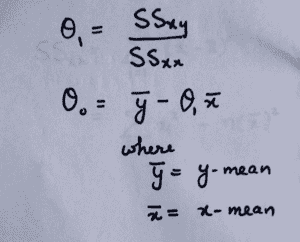Here, we are representing the features(independent variables) for each sample as x-i and their mean as x-bar. The output(dependent variables) for each sample is represented as y-i and their mean as y-bar. The total number of samples is n.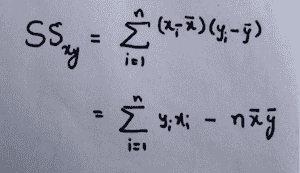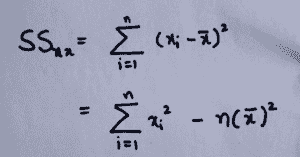After applying the above equations, we can find the best fitting line for the scattered points. The Python code for this is represented below.

import numpy as np
import matplotlib.pyplot as plt

def estimate_coef(x, y):
n = np.size(x)
m_x, m_y = np.mean(x), np.mean(y)

SS_xy = np.sum(y*x) - n*m_y*m_x
SS_xx = np.sum(x*x) - n*m_x*m_x

theta_1 = SS_xy / SS_xx
theta_0 = m_y - theta_1*m_x

return(theta_0, theta_1)

def plot_regression_line(x, y, theta):

plt.scatter(x, y, color = "b",marker = "o", s = 30)
y_pred = theta + theta*x

plt.plot(x, y_pred, color = "r")

plt.xlabel('x')
plt.ylabel('y')
plt.show()

x = np.array([0, 1, 2, 3, 4, 5, 6, 7, 8, 9])
y = np.array([11 ,13, 12, 15, 17, 18, 18, 19, 20, 22])

theta = estimate_coef(x, y)
print("Estimated coefficients:\ntheta_0 = {} \ntheta_1 = {}".format(theta, theta))

plot_regression_line(x, y, theta)

print(round(theta+ theta*11,4))

The same problem of linear regression can be solved in Machine Learning in three different ways.

The methods are:

• Using scikit-learn library’s built-in LinearRegression function.
• Using Moore-Penrose inverse method.

## Linear regression using scikit learn

The simplest method is to use a built-in library function (the code for this is given below). The dataset used is the same as the dataset used above. After fitting the line, we need to find the value of y for x = 11. We will be using the same dataset and input values for all the different methods used.

The LinearRegression() function takes the input parameters in the form of sparse matrices of shape (n_samples, n_features) and (n_samples, n_targets).

import numpy as np;
from sklearn.linear_model import LinearRegression;

x = np.array([, ,, , , , , , , ])
y = np.array([, , , , , , , , , ])

LR=LinearRegression()
LR.fit(x,y)
b=LR.predict(np.array([]))

print(round(b,4))

## Linear regression using gradient descent

Gradient Descent is one of the most common methods used to optimize different convex functions in Machine Learning. Since we know that the cost function is similar to the cost function(with a difference of a factor of 1/2) given in the Least Square Method, we will be using Gradient Descent to solve the problem. We have to minimize the cost function to find the value of Theta in the regression line.

The method of gradient descent can be represented as follows: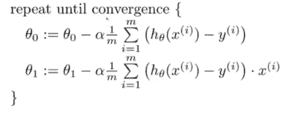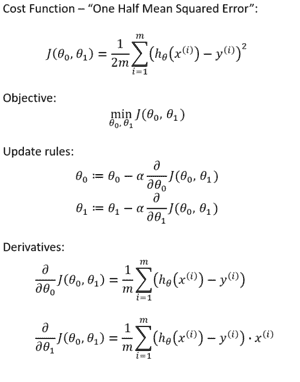Since we cannot update the values of theta-0 and theta-1 simultaneously, we use temporary variables: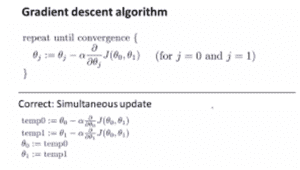import numpy as np;
from matplotlib import pyplot as plt;

# Function for cost function
def cost(z,theta,y):
m,n=z.shape;
htheta = z.dot(theta.transpose())
cost = ((htheta - y)**2).sum()/(2.0 * m);
return cost;

cost_arr=[]
m,n=z.shape;
count=0;
htheta = z.dot(theta.transpose())
while count<itr:
htheta = z.dot(theta.transpose())
a=(alpha/m)
# Using temporary variables for simultaneous updation of variables

temp0=theta[0,0]-a*(htheta-y).sum();

temp1=theta[0,1]-a*((htheta-y)*(z[::,1:])).sum();
theta[0,0]=temp0;
theta[0,1]=temp1;
cost_arr.append(float(cost(z,theta,y)));

count+=1;

cost_log = np.array(cost_arr);

plt.plot(np.linspace(0, itr, itr, endpoint=True), cost_log)
plt.xlabel("No. of iterations")
plt.ylabel("Error Function value")
plt.show()

return theta;

x = np.array([, ,, , , , , , , ])
y = np.array([, , , , , , , , , ])

m,n=x.shape;

z=np.ones((m,n+1),dtype=int);

z[::,1:]=x;

theta=np.array([[21,2]],dtype=float)

new_x=np.array([1,11])

predicted_y=new_x.dot(theta_minimised.transpose())

print(round(predicted_y,4));

## Linear regression using Pseudo inverse method

The equation for finding theta in case of Moore-Penrose inverse is:

θ = (X ′ X) −1 X ′ y

It is implemented in the code below.

import numpy as np;

# Input Matrix
x= np.array([, ,, , , , , , , ])

# Output Matrix
y= np.array([, , , , , , , , , ])
m,n=x.shape;

# Adding extra ones for the theta-0 or bias term
z=np.ones((m,n+1),dtype=int);

z[:,1:]=x; # z is Input matrix with added 1s

mat=np.matmul(z.transpose(),z); # product of z and z transpose
matinv=np.linalg.inv(mat) #inverse of above product
val=np.matmul(matinv,z.transpose()) # Product of inverse and z transpose
theta=np.matmul(val,y) # Value of theta by multiplying value calculated above to y

new_x=np.array([1,11]);
predicted_y=new_x.dot(theta);

print(round(predicted_y,4));

## Practice

Now that we’ve learned linear regression, let’s apply it to a real Dataset. The dataset we will use is the Boston dataset.

It has 506 samples, 13 features, and one column as an output column. The 14 column is output. Below is a sample code for Boston Dataset.

import numpy as np;
from sklearn.linear_model import LinearRegression;

print(X.shape)
# We can see that there are 13 features
LR=LinearRegression()
LR.fit(X,y)

# finding the price for given input
b=LR.predict([[0.00632,18.0,2.31,0.0,0.538,6.57,65.5,4.09,1,296,15.5,396.9,4.98]])

print(b)

RELATED TAGS

machine learning
community creator
data science
linear regression

CONTRIBUTORAman Anand